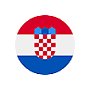# Source code of 6.1.3 - Surface of trigonometric figures```10 CLS ' Clear the screen 11 CLEAR ' Delete the contents of all variables 14 DEFDBL O 15 DEFSNG P 17 CONST cpi = 3.14592927# 20 REM Than this number further starts the program code 30 INPUT "Enter radius of circle" R 40 P1 = R ^ 2 * cpi 50 P2 = 2 * R ^ 2 60 P3 = 2 * R ^ 2 * (SIN(cpi / 3)) ^ 3 70 P4 = (P1 - P2) / 4 80 P5 = (P1 - P3) / 3 140 O1 = 2 * R * cpi 150 O2 = 4 * 2 ^ (1 / 2) * R 160 O3 = 6 * R * SIN(cpi / 3) 210 PRINT "P1=" P1 220 PRINT "P2=" P2 230 PRINT "P3=" P3 240 PRINT "P4=" P4 250 PRINT "P5=" P5 310 PRINT "O1=" O1 320 PRINT "O2=" O2 330 PRINT "O3=" O3 900 SLEEP 999 END ' Completion of the programming code 1000 REM Than this number further start sub-programs```

In line 30 requires the entry radius circle. What to do to not accept input a negative number? What would happen if instead of numbers entered a letter?

What is the difference between the display of calculated results for the perimeter and area? What command lines define these representations?

If the write line 17 as 17 CONST cpi = 3.14, disappears mark '#'. Why?Citing of this page: Radic, Drago. " IT - Informatics Alphabet " Split-Croatia. {Date of access}. . Copyright © by Drago Radic. All rights reserved. | Disclaimer